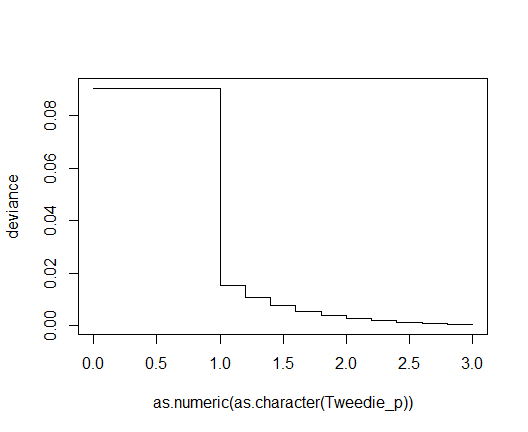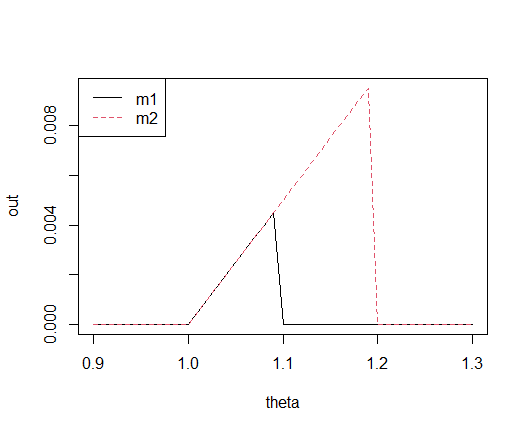# MetricsWeighted

## Overview

{MetricsWeighted} provides weighted versions of different machine learning metrics and performance measures.

They all take at least four arguments:

1. actual: Actual observed values.
2. predicted: Predicted values.
3. w: Optional vector with case weights.
4. ...: Further arguments.

## Installation

# From CRAN
install.packages("MetricsWeighted")

# Development version
devtools::install_github("mayer79/MetricsWeighted")

## Usage

### Regression

library(MetricsWeighted)

# The data
y_num <- iris[["Sepal.Length"]]
fit_num <- lm(Sepal.Length ~ ., data = iris)
pred_num <- fit_num$fitted weights <- seq_len(nrow(iris)) # Performance metrics rmse(y_num, pred_num) #>  0.300627 rmse(y_num, pred_num, w = rep(1, length(y_num))) # same #>  0.300627 rmse(y_num, pred_num, w = weights) # different #>  0.3138009 mae(y_num, pred_num) #>  0.2428628 medae(y_num, pred_num, w = weights) #>  0.2381186 # MSE = mean normal deviance = mean Tweedie deviance with p = 0 mse(y_num, pred_num) #>  0.09037657 deviance_normal(y_num, pred_num) #>  0.09037657 deviance_tweedie(y_num, pred_num, tweedie_p = 0) #>  0.09037657 # Mean Poisson deviance equals mean Tweedie deviance with parameter 1 deviance_poisson(y_num, pred_num) #>  0.01531595 deviance_tweedie(y_num, pred_num, tweedie_p = 1) #>  0.01531595 # Mean Gamma deviance equals mean Tweedie deviance with parameter 2 deviance_gamma(y_num, pred_num) #>  0.002633186 deviance_tweedie(y_num, pred_num, tweedie_p = 2) #>  0.002633186 ### Binary classification # The data y_cat <- iris[["Species"]] == "setosa" fit_cat <- glm(y_cat ~ Sepal.Length, data = iris, family = binomial()) pred_cat <- predict(fit_cat, type = "response") # Performance metrics AUC(y_cat, pred_cat) # unweighted #>  0.9586 AUC(y_cat, pred_cat, w = weights) # weighted #>  0.9629734 logLoss(y_cat, pred_cat) # Log loss = binary cross-entropy #>  0.2394547 deviance_bernoulli(y_cat, pred_cat) # Log Loss * 2 #>  0.4789093 ### Generalized R-squared Furthermore, we provide a generalization of R-squared, defined as the proportion of deviance explained, i.e., one minus the ratio of residual deviance and intercept-only deviance, see . For out-of-sample calculations, the null deviance is ideally calculated from the average in the training data. This can be controlled by setting reference_mean to the (possibly weighted) average in the training data. summary(fit_num)$r.squared
#>  0.8673123

# Same
r_squared(y_num, pred_num)
#>  0.8673123
r_squared(y_num, pred_num, deviance_function = deviance_tweedie, tweedie_p = 0)
#>  0.8673123

### Pipe

In order to facilitate the use of these metrics with the pipe, use the function performance(): Starting from a data set with actual and predicted values (and optional case weights), it calculates one or more metrics. The resulting values are returned as a data.frame.

library(dplyr)

fit_num <- lm(Sepal.Length ~ ., data = iris)

# Regression with Sepal.Length as response
iris %>%
mutate(pred = predict(fit_num, data = .)) %>%
performance("Sepal.Length", "pred")

>  metric    value
>    rmse 0.300627

# Multiple measures
iris %>%
mutate(pred = predict(fit_num, data = .)) %>%
performance(
"Sepal.Length",
"pred",
metrics = list(rmse = rmse, mae = mae, R-squared = r_squared)
)

>    metric     value
>      rmse 0.3006270
>       mae 0.2428628
> R-squared 0.8673123

### Parametrized scoring functions

Some scoring functions depend on a further parameter $$p$$:

• tweedie_deviance(): depends on tweedie_p.
• elementary_score_expectile(), elementary_score_quantile(): depend on theta.
• prop_within(): Depends on tol.

It might be of key relevance to evaluate such function for varying $$p$$. That is where the function multi_metric() shines.

ir <- iris
ir\$pred <- predict(fit_num, data = ir)

# Create multiple Tweedie deviance functions
multi_Tweedie <- multi_metric(deviance_tweedie, tweedie_p = c(0, seq(1, 3, by = 0.2)))
perf <- performance(
ir,
actual = "Sepal.Length",
predicted = "pred",
metrics = multi_Tweedie,
key = "Tweedie_p",
value = "deviance"
)
#>   Tweedie_p    deviance
#> 1         0 0.090376567
#> 2         1 0.015315945
#> 3       1.2 0.010757362
#> 4       1.4 0.007559956
#> 5       1.6 0.005316008
#> 6       1.8 0.003740296

# Deviance against p
plot(deviance ~ as.numeric(as.character(Tweedie_p)), data = perf, type = "s")### Murphy diagrams

The same logic as in the last example can be used to create so-called Murphy diagrams . The function murphy_diagram() wraps above calls and allows to get elementary scores for one or multiple models across a range of theta values, see also R package murphydiagram.

y <- 1:10
two_models <- cbind(m1 = 1.1 * y, m2 = 1.2 * y)
murphy_diagram(y, two_models, theta = seq(0.9, 1.3, by = 0.01))